# Texas Go Math Grade 3 Lesson 6.3 Answer Key Model Multiplication

Refer to our Texas Go Math Grade 3 Answer Key Pdf to score good marks in the exams. Test yourself by practicing the problems from Texas Go Math Grade 3 Lesson 6.3 Answer Key Model Multiplication.

## Texas Go Math Grade 3 Lesson 6.3 Answer Key Model Multiplication

Unlock the Problem

Three groups of students are taking drum lessons. There are 8 students in each group. How many students are taking lessons in all?

What do I need to find?

I need to find how many students are taking drum lessons.

What information am I given?

There are 3 groups of students taking drum lessons. There are 8 students in each group.

Plan

What is my plan or strategy?

I will draw a strip diagram to help me see

Solve

Complete the strip diagram to show the drummers.

Write 8 in each box to show the 8 students in each of the 3 groups.Since there are equal groups I can multiply to find the number of students taking lessons.
________ × _________ = ☐
________ = ☐
So, there are __________ students in all.
3 x 8 = 24 students
So, there are 24 students in all.
Explanation:
Three groups of students are taking drum lessons.
There are 8 students in each group.
Number of students taking lessons in all
3 x 8 = 24

Math Talk
Mathematical Processes

How would the strip diagram change if there were 6 groups of students? Solve.
48 students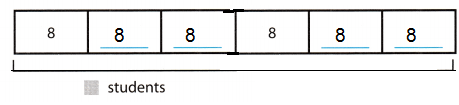6 x 8 = 48
Explanation:
A strip diagram is usually drawn as a rectangle that can be partitioned into different sized parts where each part may refer to a quantity.
3 more groups of students to be added to show 6 groups of students.

Try Another Problem

I will draw a strip diagram to help me see

Total 12 students and 7 for drums, rest made 2 maracas. How many maracas in all did the students make?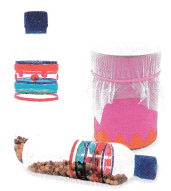What do I need to find?
Students of maracas in all were made.

What information am I given?
Total students are 12
7 are for drums,

Plan

What is my plan or strategy?

I will draw a strip diagram to find the solution.
Solve

Record the steps you used to solve the problem.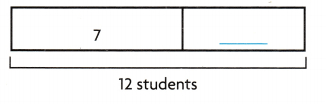Question 1.
How many maracas in all did the students make?
5 students
Explanation:
Total students 12
Drums students 7
12 – 7 = 5
5 students maracas

Question 2.
Yes, my answer is reasonable, as i have the complete data.
Explanation:
Total students 12
Drums students 7
12 – 7 = 5
5 students maracas

Math Talk
Mathematical Processes

Why wouldn’t you draw 2 boxes and write 5 in each box?
As there is no equal group of students
Explanation:
Total 7 students in drums.
Total 5 students for maracas.

Unlock the Problem
Use the Problem Solving Math Board.
Underline important facts.
Choose a strategy you know.

Share and Show

Question 1.
There are 6 groups of 4 students who play the trumpet in the marching band. How many students play the trumpet in the band?
First, draw a strip diagram to show each group of students.
Draw ________ boxes and write __________ in each box.Then, multiply to find the total number of trumpet players.
________ × _________ = ☐
________ = ☐
So, _________ students play the trumpet.
Draw 6 boxes and write 4 in each box.6  x 4 = 24
Explanation:
A strip diagram is usually drawn as a rectangle that can be partitioned into different sized parts where each part may refer to a quantity.
6 boxes and 4 groups in each box.

Question 2.
What if there are 4 groups of 7 students who play the trumpet? How many students play the trumpet?4 x 7 = 28 play the trumpet
Explanation:
There are 4 groups of 7 students who play the trumpet.
Number of students play the trumpet
4 x 7 = 28

Problem Solving

Question 3.
H.O.T. Multi-Step Suppose there are 5 groups of 4 trumpet players. In front of the trumpet players are 18 saxophone players. How many students play the trumpet or saxophone?38 students
Explanation:
trumpet players = 5 x 4 = 20
saxophone players = 18
Total students play the trumpet or saxophone = 20 + 18 = 38

Question 4.
H.O.T. Evaluate Zoey plays soccer. The players on her team have the numbers 1 to 11 on their uniforms. Zoey’s uniform has a number greater than 6. You can start with 4 and skip count by fours to find her number. What is Zoey’s number?
Zoey’s number is 8
Explanation:
The players on her team have the numbers 1 to 11 on their uniforms.
Zoey’s uniform has a number greater than 6.
Start with 4 and skip count by fours, then Zoey’s number is 8Fill in the bubble for the correct answer choice. You can use a strip diagram to solve the problems.

Question 5.
There are 6 boxes of sidewalk chalk in the activity center. Each box has 5 pieces of chalk. How many pieces of chalk are there altogether?
(A) 35
(B) 30
(C) 11
(D) 20
Option (B)
Explanation:
There are 6 boxes of sidewalk chalk in the activity center.
Each box has 5 pieces of chalk.
Total pieces of chalk altogether
6 x 5 = 30

Question 6.
Analyze Greg plants bean seeds in 4 rows. Each row has 9 bean seeds. How many bean seeds are there?
(A) 13
(B) 32
(C) 45
(D) 36
Option (D)
Explanation:
Analyze Greg plants bean seeds in 4 rows.
Each row has 9 bean seeds.
Number of bean seeds 4 x 9 = 36

Question 7.
Multi-Step Savannah has 2 baskets. Each basket holds 10 pieces of fruit. Four pieces of fruit in each basket are apples, and the rest are oranges. How many oranges does Savannah have?
(A) 16
(B) 8
(C) 12
(D) 10
Option (C)
Explanation:
Each basket holds 10 pieces of fruit.
Four pieces of fruit in each basket are apples = 4 x 2 = 8
Number of oranges Savannah have 6 x 2 = 12

Texas Test Prep

Question 8.
After music class, the students filled 4 cabinets with their instruments. Each cabinet has space for 8 instruments. How many instruments did the students put away?
(A) 32
(B) 16
(C) 24
(D) 12
Option (A)
Explanation:
4 cabinets and each cabinet has space for 8 instruments
number of instruments students put away
4 x 8 =32

### Texas Go Math Grade 3 Lesson 6.1 Homework and Practice Answer Key

Question 1.
Dale has 3 baskets. He puts 9 apples in each basket. How many apples does Dale have?27 apples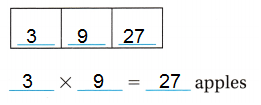Explanation:
He puts 9 apples in each basket.
Number of apples Dale have
3 x 9 = 27

Question 2.
Hans has 6 boxes of crayons. Each box has 5 crayons. How many crayons does Hans have?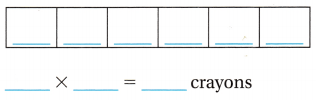30 crayonsExplanation:
Hans has 6 boxes of crayons.
Each box has 5 crayons.
Number of crayons Hans have
6 x 5 = 30

Question 3.
In art class, there are 7 tables with 4 students at each table. How many students are in art class?
28 students
Explanation:
In art class, there are 7 tables with 4 students at each table.
Number of students in art class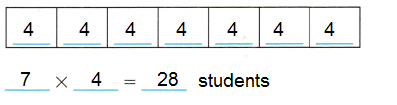Question 4.
In gym class there are 6 teams with 4 students on each team. How many students are in gym class?
24 students
Explanation:
In gym class there are 6 teams with 4 students on each team.
Number of students in gym classProblem Solving

Question 5.
Nine students in Mr. Dobb’s class work on an art project. Six students paint a picture. The rest make 3 puppets each. How many puppets are made?
What do you need to find? Write a number sentence to solve.
9 puppets.
Explanation:
Nine students in Mr. Dobb’s class work on an art project.
Six students paint a picture.
9 – 6 = 3
The rest make 3 puppets each.
3 x 3 = 9

Question 6.
Ten students in Mrs. Porth’s class bring muffins to the bake sale. Six students bring bran muffins. The rest bring 6 blueberry muffins each. How many blueberry muffins do the students bring?
What do you need to find? Write a number sentence to solve.
4 students brought blueberry muffins.
Explanation:
Ten students in Mrs. Porth’s class bring muffins to the bake sale.
Six students bring bran muffins.
10 – 6 = 4
The rest bring 6 blueberry muffins each.
Total blueberry muffins the students brought = 6
I need to find number of students i.e., 4
Number sentence 10 – 6 = 4

Lesson Check

Question 7.
Ms. Cabrera has 4 rows of tomato plants in her garden. Each row has 7 plants. How many tomato plants does Ms. Cabrera have?
(A) 16
(B) 21
(C) 28
(D) 11
Option (B)
Explanation:
Ms. Cabrera has 4 rows of tomato plants in her garden.
Each row has 7 plants.
Number of tomato plants Ms. Cabrera have
4 x 7 = 21

Question 8.
Mr. Myers has 5 packs of markers. Each pack has 5 markers. How many markers does Mr. Myers have?
(A) 10
(B) 25
(C) 55
(D) 20
Option (B)
Explanation:
Mr. Myers has 5 packs of markers.
Each pack has 5 markers.
Total markers Mr. Myers have
5 x 5 = 25

Question 9.
At the farm, there are 3 stables. There are 8 horses in each stable. How many horses are at the farm?
(A) 38
(B) 11
(C) 24
(D) 27
Option (C)
Explanation:
At the farm, there are 3 stables.
There are 8 horses in each stable.
Total horses at the farm
8 x 3 = 24

Question 10.
Alyssa has 4 boxes. In each box there are 8 shells. How many shells does Alyssa have?
(A) 32
(B) 28
(C) 12
(D) 48
Option (A)
Explanation:
Alyssa has 4 boxes.
In each box there are 8 shells.
Total shells Alyssa have
4 x 8 = 32

Question 11.
Multi-Step Alex has 2 bunches of flowers. There are 9 flowers in each bunch. Five of the flowers are daises and the rest are tulips. How many flowers are tulips?
(A) 18
(B) 16
(C) 45
(D) 13
Option (D)
Explanation:
Alex has 2 bunches of flowers.
There are 9 flowers in each bunch.
Total flowers  in 2 bunches = 9 x 2  = 18
Five of the flowers are daises and the rest are tulips.
Total tulips = 18 – 5 =13

Question 12.
Multi-Step In a garden there are 3 rows of plants. There are 5 plants in each row. Six of the plants are pumpkin plants and the rest are corn. How many corn plants are in the garden?
(A) 15
(B) 35
(C) 9
(D) 11